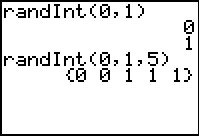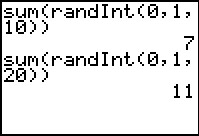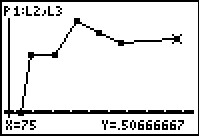••• ##### Device
• TI-83 Plus Family
• TI-84 Plus
• TI-84 Plus Silver Edition
•TI-84 Plus C Silver Edition
•TI-84 Plus CE

# Statistics: To Toss or To Simulate?

by Texas Instruments - Bell Ringer Lesson#### Overview

Students use the RandInt() command to simulate the tossing of a coin and the rolling of a die. They will collect data for several trials and use the information to investigate the Law of Large Numbers.

#### Key Steps

•In this activity, students are introduced to using the Random Integer command to simulate an experiment. First, they will explore the difference between entering the command randInt(0,1) five times and entering randInt(0,1,5).

•Then students will use the randInt command in a list to simulate the tossing of a coin for a various number of trials. The sum command will help them count the number of heads for a simulation.

•After all the data has been collected, students will calculate the percent of heads and create a scatter plot of percent of heads versus number of tosses. They can graph the theoretical probability as a horizontal line for comparison.

The goal is to see that the experimental probability will approach the theoretical probability when the number of trials is large.